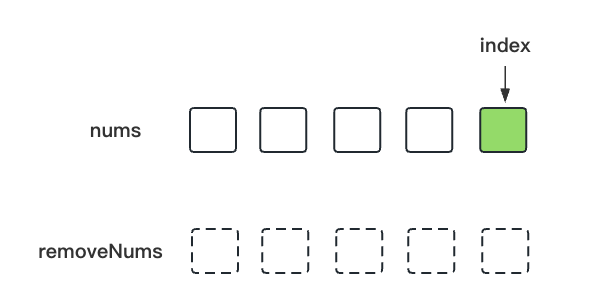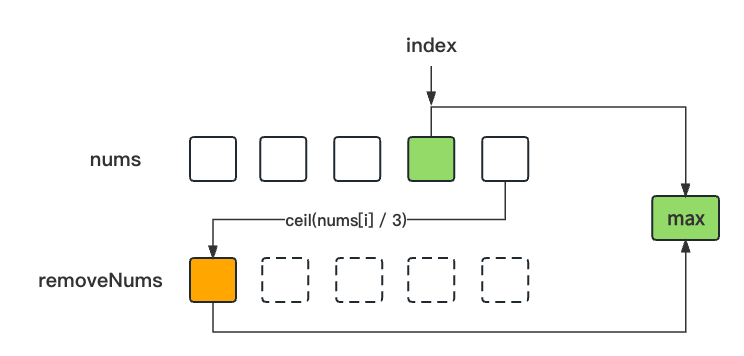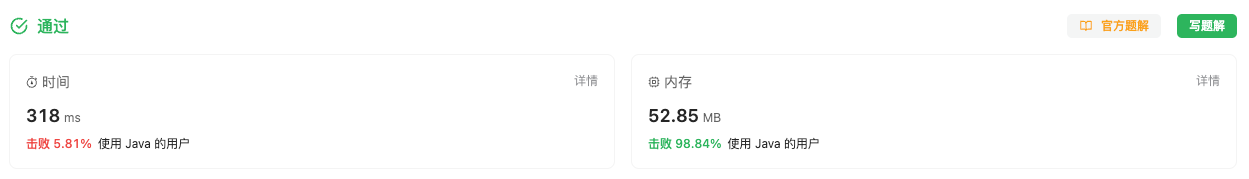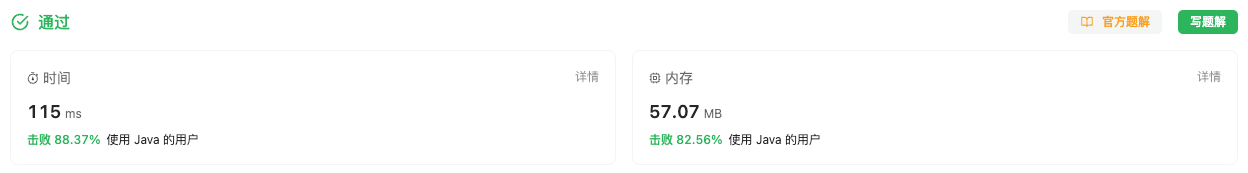#### 2530. 执行 K 次操作后的最大分数

###### 来源: 每日一题 2023.10.18

`nums[i]` 替换为 `ceil(nums[i] / 3)`

``````输入：nums = [10,10,10,10,10], k = 5

``````

``````输入：nums = [1,10,3,3,3], k = 3

``````

• `1 <= nums.length, k <= 10^5`
• `1 <= nums[i] <= 10^9`
``````class Solution {
public long maxKelements(int[] nums, int k) {

}
}
``````

#### 分析与题解

• 暴力求解 时间超时, 失败

直接暴力法就是我们按照题意进行模拟运行, 每一次取完数组中的最后一个值就根据题意更新这个值, 然后重新排序整个数组.

``````long result = 0;
for(int i = 0; i < k; i++) {
int num = nums[nums.length - 1];
result += num;
num = num/3 + (num%3 > 0 ? 1 : 0);
nums[nums.length - 1] = num;
Arrays.sort(nums);
}
``````

整体的解题思路如下所示.

``````class Solution {
public long maxKelements(int[] nums, int k) {
Arrays.sort(nums);
long result = 0;
for(int i = 0; i < k; i++) {
int num = nums[nums.length - 1];
result += num;
num = num/3 + (num%3 > 0 ? 1 : 0);
nums[nums.length - 1] = num;
Arrays.sort(nums);
}
return result;
}
}
``````

复杂度分析:

• 时间复杂度: O(klogn), 时间复杂度与`k`相关, 排序需要损耗的时间复杂度为 `logn`
• 空间复杂度: O(klogn), 需要 k 次排序, 每一次都要消耗的空间复杂度为 `logn`

结果如下所示.• 额外数组法

既然暴力排序的方式时间超时, 其原因就在于`Arrays.sort(nums);`, 那我们就找一个不用排序的方式.

我们发现, 当我们对 `nums` 排序完成之后, 然后取出最后一位数据时, 并进行 `ceil(nums[i] / 3)`, 生成许多的 `item`, 一定有这样的规律: 先生成的 `item` 一定不小于后生成的 `item` 的值.

比如 `[1, 10, 3, 3, 3]` 第一次是 `ceil(10 / 3) = 4`, 第二次是 `ceil(4 / 3) = 2`, 第三次是 `ceil(3 / 3) = 1`, 结果是 `4 > 2 > 1`, 结论是成立的.

我们把这些 `ceil(nums[i] / 3)` 生成的 `item` 单独并且依次放在一个数组`removeNums`中, 那么这个数组`removeNums`也是有序的.

由于 `nums` 不再删除数据了, 所以我们要用一个 `index` 记录当前 `nums` 中能取到的最大值.

这时候取值过程就要分不同的逻辑分支了.

• `removeNums` 没有数据时, 我们就从 `nums` 中取最大值. 同时, index也需要偏移. 并且把 `ceil(nums[i] / 3)` 添加到 `removeNums` 中.``````int num = nums[numsIndex];
result += num;
removeNums.add(num/3 + (num%3 > 0 ? 1 : 0));
numsIndex--;
``````
• `removeNums` 有数据时, 我们就从 `nums` 中取最大值 和 从 `removeNums` 中取最大值, 两者取较大值. 当然了这时候还是需要更新 `removeNums` 的数据(删除最大值, 计算后放入数组尾部).``````int num = removeNums.get(0);
removeNums.remove(0);
result += num;
removeNums.add(num/3 + (num%3 > 0 ? 1 : 0));
``````

接下来, 我们就一起看一下按照这个思路的整体的解题过程.

``````class Solution {
public long maxKelements(int[] nums, int k) {
Arrays.sort(nums);
long result = 0;
ArrayList<Integer> removeNums = new ArrayList<>();
int numsIndex = nums.length - 1;
for(int i = 0; i < k; i++) {
// 条件1: 如果 numsIndex 小于等于0
// 条件2: 如果当前removeNums有数据, 并且当前的removeNums的数据大于nums[numsIndex], 那就从removeNums中取出数据
// numsIndex 下标大于等于0是边界条件
if( numsIndex < 0 || (removeNums.size() != 0 && removeNums.get(0) > nums[numsIndex])) {
int num = removeNums.get(0);
removeNums.remove(0);
result += num;
removeNums.add(num/3 + (num%3 > 0 ? 1 : 0));
} else {
int num = nums[numsIndex];
result += num;
removeNums.add(num/3 + (num%3 > 0 ? 1 : 0));
numsIndex--;
}
}
return result;
}
}
``````

复杂度分析:

• 时间复杂度: O(k + logn), 遍历时间复杂度与`k`相关, 排序需要损耗的时间复杂度为 `logn`
• 空间复杂度: O(logn), 排序消耗的空间复杂度为 `logn`

结果如下所示.• 优先队列法

直接使用Java内置的优先队列 `PriorityQueue`, 这种方式我们不需要管理内部的排序逻辑, 直接通过 `PriorityQueue`来完成.

首先就是把所有的数据添加到优先队列中.

``````PriorityQueue<Integer> q = new PriorityQueue<Integer>((a, b) -> b - a);
for (int num : nums) {
q.offer(num);
}
``````

然后就是通过 `poll()` 取出最大值, 然后计算后再添加到优先队列中.

``````long ans = 0;
for (int i = 0; i < k; ++i) {
int num = q.poll();
ans += num;
q.offer(num/3 + (num%3 > 0 ? 1 : 0));
}
``````

接下来, 我们一起看一下整体的代码逻辑.

``````class Solution {
public long maxKelements(int[] nums, int k) {
PriorityQueue<Integer> q = new PriorityQueue<Integer>((a, b) -> b - a);
for (int num : nums) {
q.offer(num);
}
long ans = 0;
for (int i = 0; i < k; ++i) {
int num = q.poll();
ans += num;
q.offer(num/3 + (num%3 > 0 ? 1 : 0));
}
return ans;
}
}
``````

复杂度分析:

• 时间复杂度: O(nk), 遍历时间复杂度与`k` `nums的长度` 相关
• 空间复杂度: O(n), 优先队列消耗的空间复杂度为 `O(n)`

结果如下所示.IT界无底坑洞栋主 欢迎加Q骚扰:676758285